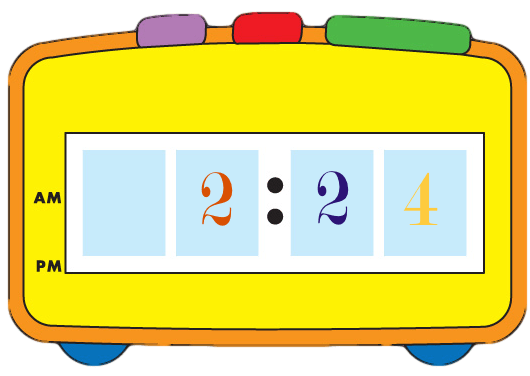# Level 1 Content

Students are asked to categorize linear, square, and cubic units as either distance, area, or volume and to count and compute total amounts of each.Students are asked to tell and write time by counting the hour and the minutes before and after the hour on both digital and analogue clocks.Students are asked to identify money as either pennies, nickels, dimes, quarters, half dollars, or dollars. Penny equivalents of each are examined and total amounts are counted and written down with dollar and cent notation.